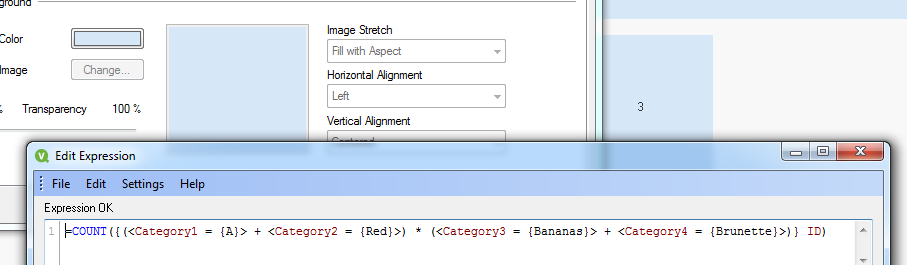# QlikView App Development

Discussion Board for collaboration related to QlikView App Development.

Highlighted
Contributor

## Combining Set Analysis Operators

Hi,

I know that to create a union between two sets you use syntax such as

<set1> + <set2>

and to create the intersection of twos sets you use

<set1> * <set2>

But is there any was of combining these operators?

For instance I would like to create the intersection of sets, each of which is the union of two sets itself

e.g.

(<set1> + <set2>) * (<set3> + <set4>)

Doesn't like the parenthesis though

Any ideas?

Matt

Tags (2)
1 Solution

Accepted SolutionsMVP

## Re: Combining Set Analysis Operators

This seems to work:

=COUNT({(<Category1 = {A}> + <Category2 = {Red}>) * (<Category3 = {Bananas}> + <Category4 = {Brunette}>)} ID)10 RepliesMVP

## Re: Combining Set Analysis Operators

Seems to work for me.

Could you upload a sample that doesn't work as expected (and maybe add the expected result, too)?

edit:

Tested on QV12 IR with set expressions likePartner

## Re: Combining Set Analysis Operators

Hi,

Not sure what your problem is but use of parenthesis is covered by manual:

sum( {\$<Year = Year + ({“20*”,1997} – {2000}) >} Sales )

sum( {\$<Year = (Year + {“20*”,1997}) – {2000} >} Sales )

These two expressions have different logic due to use of parenthesis.

Best regards,

MaximMVP

## Re: Combining Set Analysis Operators

What exactly are your sets, may be the two sets don't have anything in common and that's why you don't see any results?

Contributor

## Re: Combining Set Analysis Operators

Thanks for the responses everyone. How do I upload a qlikview file to the forum? I've put together a simplified exampleMVP

Contributor

## Re: Combining Set Analysis Operators

Here we go...

Here's a simplified example of what I'm trying to do. The first two combined sets (Union) work as expected, then what I want to do is to get the intersection of these two combined sets, but the result isn't as expected, I would expect the and (Count) to be 3 (ids 4, 8 and 10).

Cheers

MattMVP

## Re: Combining Set Analysis Operators

This seems to work:

=COUNT({(<Category1 = {A}> + <Category2 = {Red}>) * (<Category3 = {Bananas}> + <Category4 = {Brunette}>)} ID)MVP

## Re: Combining Set Analysis Operators

Neverming the red wiggly lines underneath set expression parts in aggregation expressions, if that is what is bothering you. In many cases, even the syntax checker in the expression editor isn't aware of what QlikView is capable of.

P.MVP

## Re: Combining Set Analysis Operators

I don't even get red wiggly lines.

Matt, I believe you should get your expected result if you use parentheses as you indicated in your OP (but it seems you've removed these from your sample file).

=COUNT({(<Category1 = {A}> + <Category2 = {Red}>) * (<Category3 = {Bananas}> + <Category4 = {Brunette}>)} ID)

You will get an error if you place the set identifier at the wrong place:

Error:

=COUNT({\$(<Category1 = {A}> + <Category2 = {Red}>) * (<Category3 = {Bananas}> + <Category4 = {Brunette}>)} ID)

While this should be ok (or just remove the default set identifier:

=COUNT({(\$<Category1 = {A}> + \$<Category2 = {Red}>) * (\$<Category3 = {Bananas}> + \$<Category4 = {Brunette}>)} ID)